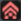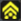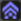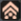If you are looking for Information about PSU Clementine, Go check their Wiki

# Formulae

This page will contain all the various formulae found in the game. Those formulae dictate how the game works and have been proven through various means.

## Buff boosts

Buffs give the following boost to your attributes:

Type Attr. LV1 LV2 LV3 LV4 LV5ATP 111% 115% 119% 123% 127%ATADFPTP

Those values are not confirmed and are likely only approximations.

## Physical damage formula

First you have to calculate your total ATP:

`atp = character's atp + line shield's atp + unit's atp + weapon's atp`

Then get your PA modifier value correctly. It's a percent, therefore:

`pa = pa modifier / 100`

You can get the buff boost for ATP by looking at the above table and dividing by 100. If you used an agtaride, then `buff = 1.15`.

To determine the elemental modifier you must first ask yourself whether you attack with the correct element. If you attack with neutral, or an element different than the enemy's element or its opposite element, then `ele = 0`. Otherwise, apply the following formula to obtain the element modifier:

`ele = (5 + element's %) / 151.5`

If you used the same element as the enemy then multiply ele by -1.

If the hit is critical then `crit = 1.5`, otherwise `crit = 1`.

You can find the enemy's DFP by first looking at the enemies' page and then multiplying that DFP by the modifier found on each enemy's page.

Knowing all those variables, you can now calculate the maximum damage you will do using physical attacks:

`maximum damage = floor(crit x (atp x pa x (1 + ele) x buff - enemy's dfp) / 5)`

This value is the maximum damage you can make in these settings. To obtain the complete damage range your weapon can make you must apply the variance.

## TECHNICs damage formula

This formula works pretty much like the above formula, with a few exceptions. For the sake of convenience only the exceptions will be listed.

Instead of ATP you have TP, but you calculate the total TP in the same way:

`tp = character's tp + line shield's tp + unit's tp + weapon's tp`

The element modifier's calculation is different. TECHNICs get a lot of boost on this point, so using the correct element is really important. If you use the wrong element then `ele = 0`. Otherwise it is dependent on the weapon's elemental percent and the PA level you're using:

`ele = (14 + element's % + PA's level) / 151.5`

If you used the same element as the enemy then multiply ele by -1.

If you use a rod then you get a boost and `rod = 1.2`. Otherwise `rod = 1`.

You also need to know the PA modifier, buff boost (this time for TP) and critical value, defined above.

The resulting formula for the maximum damage using TECHNICs is:

`maximum damage = floor(crit x (rod x tp x pa x (1 + ele) x buff - enemy's mst) / 5)`

This value is the maximum damage you can make in these settings. To obtain the complete damage range your weapon can make you must apply the variance.

## Variance

A weapon's damage have a minimal and maximal value. The variance exists because every weapon in the game have a high ATP value (shown in its stats) and low ATP value (invisible). The low ATP value is obtained by substracting the variance from the high ATP value. The variance do not change between grinds, only between weapons. The variance cannot cause the weapon ATP to reach below 1.

`low ATP = high ATP - variance`

The average ATP can be calculated with the following simple formula:

`average ATP = high ATP - variance / 2`

If you know the low ATP value of a weapon you can calculate its minimum damage by applying the above formula and replacing the weapon ATP by its lower ATP.

The actual damage on the other hand is a random number between the minimum and maximum values, obtained through this formula:

`actual damage = random(minimum damage, maximum damage)`

with `random(x, y) = a random number between x and y included`.

Note that in the case of critical hits, the minimum and maximum damage calculated both are critical hits.

The variance's precision is of more or less 5 ATP. We cannot calculate it better than this because of the way PSU calculates the damage done on enemies.

## Hit rate formula

First you need to be aware that TECHNICs never miss. If you're not using a TECHNIC, proceed with the formula.

You need to know the enemy's EVP. You can find it by first looking at the enemies' page and then multiplying that EVP by the modifier found on each enemy's page.

Then you need to calculate your total ATA which is:

`ata = character's ata + line shield's ata + unit's ata + weapon's ata`

Then get your PA modifier value correctly. Make sure to use the value for accuracy. It's a percent, therefore:

`pa = pa modifier / 100`

The resulting hit rate can be calculated with this simple formula:

`hit rate = 1 - enemy's evp / (ata x pa modifier x 10)`

You can multiply this result by 100 to obtain a percent value.

 Gameplay Photon arts | Skills | Bullets | TECHNICs | Item synthesis | Weapon grinding | Set bonuses | Partner machines | Elements | Status effects | Stats | Formulae | Enemies | Enemy spawn charts | Photon Fortune